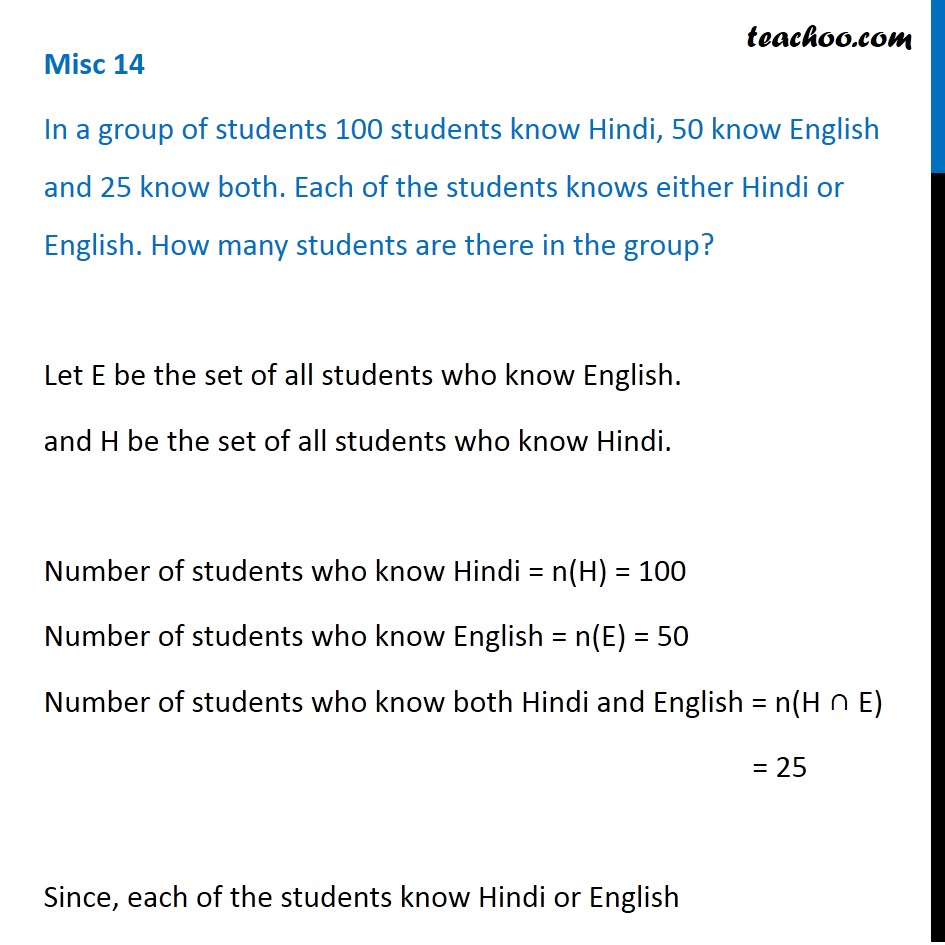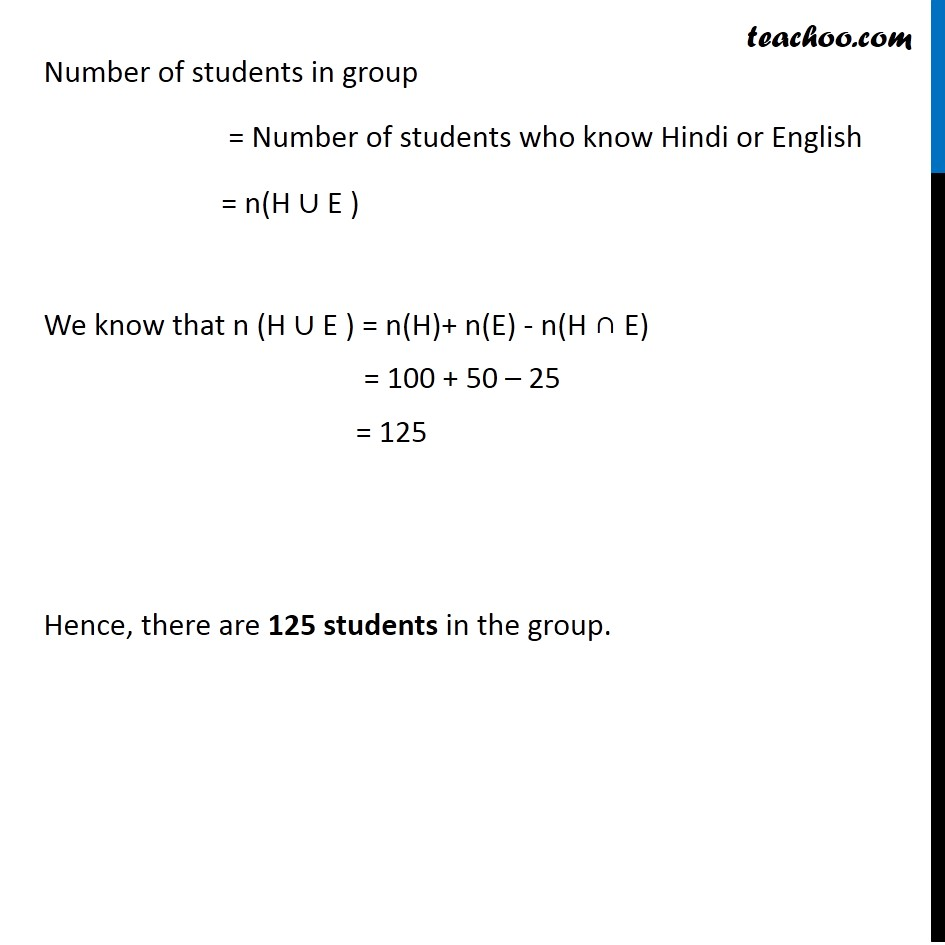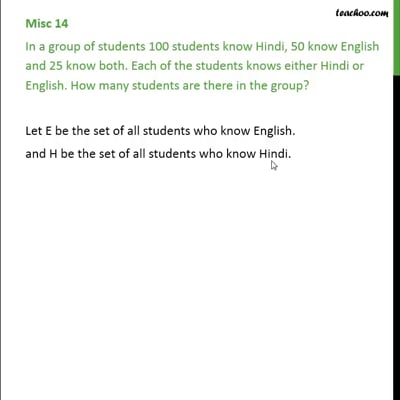Miscellaneous

Chapter 1 Class 11 Sets
Serial order wiseThis video is only available for Teachoo black users

Solve all your doubts with Teachoo Black (new monthly pack available now!)

### Transcript

Misc 14 In a group of students 100 students know Hindi, 50 know English and 25 know both. Each of the students knows either Hindi or English. How many students are there in the group? Let E be the set of all students who know English. and H be the set of all students who know Hindi. Number of students who know Hindi = n(H) = 100 Number of students who know English = n(E) = 50 Number of students who know both Hindi and English = n(H ∩ E) = 25 Since, each of the students know Hindi or English Number of students in group = Number of students who know Hindi or English = n(H ∪ E ) We know that n (H ∪ E ) = n(H)+ n(E) - n(H ∩ E) = 100 + 50 – 25 = 125 Hence, there are 125 students in the group.